# Subtractive Color Mixture Computation (Page 2)

#### Mathematics of Human Color Perception

Our eyes are sensitive to a range of electromagnetic radiation oscillating faster than radio waves and microwaves, but slower than x-rays and gamma rays. The wavelength range of visible light is roughly 400 to 700 nanometers (nm) going from the violet end to the red end of the spectrum.

Generally, we can describe how an object appears to have a color as a four step process.

1. The object is illuminated by a light source.
2. The object selectively reflects light according to its reflectance properties.
3. The reflected light, or stimulus, passes from the object and into our eyes.
4. The stimulus is processed by our brain to give the impression of a colored object.

Let’s look at each step in more detail:

##### Light Source

A light source has various levels of power at each frequency that can be summarized in a plot of relative power vs frequency, for example, as shown in the figure.

This is one of the “standard” illuminants used in colorimetric studies, called D65. It resembles daylight and is the reference illuminant for the sRGB color space (more on that later). Notice how it peaks more toward the blue end of the spectrum, making it a “cooler” light source than indoor incandescent illumination, which has much more power on the red end of the spectrum.

##### Reflectance Curve

The reflectance properties of an object are conveniently summarized in a reflectance curve, which describes the fraction of incoming light that is reflected at each wavelength. This can be measured using a spectrophotometer. For example, the reflectance curve shown in the figure was measured from a bright red object.

Notice how this red sample reflects around 70% of the longer wavelengths, and very little of the shorter wavelengths.

Since reflectance is a fraction of reflected light, it falls in the range 0 to 1 at each wavelength. The only exception to this is with fluorescent objects, which can reflect more that 100% of incoming light at certain wavelengths, and therefore, have some reflectance values greater than 1.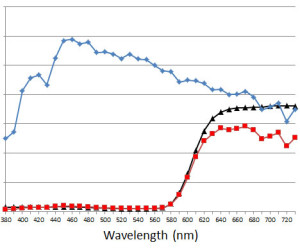The final light stimulus (red) is the product of the illuminant (blue) and the reflectance (black).

##### Stimulus

To get the distribution of light that enters our eyes (the “stimulus“), we simply multiply the illuminant by the reflectance, as shown by the red curve in this figure.

##### Color Sensation

The last step is to describe mathematically how our brain interprets a color stimulus, providing us with a color sensation. It can be described by a fairly simple matrix equation:where,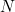is the stimulus vector with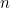values,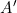is a 3x“color matching functions” matrix,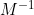is a 3×3 conversion matrix between “tristimulus values” and “linear RGB” values,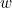is a normalizing factor that is related to the illuminant, and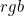is the 3×1 RGB description of the color before gamma correction, or the “linear RGB” values.

More Details...
The three rows of thematrix comprise three “color matching functions,” describing the trichromatic nature of human color vision. They were determined experimentally in several studies over the past century. One in particular, published in 1931, has become the standard model of human color vision. Here is a text file of thematrix. It summarizes how a human observer would mix three specified primary colored lights to visually match a fourth target color. Whenis multiplied by the stimulus vector,, and divided by a normalizing factor,, a three-valued color specification is produced, in this case, a set of “tristimulus values,”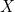,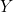, and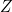.

The normalizing factor,, is simply the scalar product of the illuminant vector and the second row of. This makes the tristimulusvalue equal to 1 when the stimulus vector equals the illuminant. The handy thing about normalizing in this way is that the particular units of the stimulus vector no longer matter because they are cancelled out by the normalizing factor.

The tristimulus values,,, andare converted to linear rgb values through a linear transformation matrix,. There are many RGB color spaces, and each has its own RGB “primaries” and reference illuminant (or “white point”). In the case of the sRGB color space (which is referenced to illuminant D65), thematrix has the values shown here.

Note that I’m using the symbolhere; the standard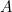matrix isx3 instead of 3x, and soindicates that it has been transposed.

This can be further simplified for the special case of obtaining linear RGB values,, directly from a reflectance curve,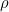. The,, andterms can all be combined together ahead of time into a single matrix,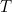. Furthermore, sinceis the product of the illuminant and the reflectance curve,, the illuminant can be extracted fromand also be combined into thematrix, leaving only the reflectance curve behind: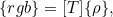whereis a vector of reflectance values in the range 0 to 1.

More Details...
The stimulus,, can be expressed as the product of a diagonal illuminant matrix,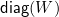, and a reflectance vector,. Putting the illuminant on the diagonal of a matrix will yield a stimulus vector of the proper shape when multiplied by the reflectance vector,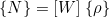. Now, when the stimulus is expressed this way, it is evident that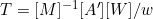.

The size ofand the length ofdepend on how finely we measure the reflectance curve. Typically, it is measured every 10 nm in the range 380 nm to 730 nm, makinga 3×36 matrix anda 36×1 vector. Here are the specific values for thematrix (again, for the special case of D65-referenced linear RGB calculation from a reflectance curve).

More Details...
If you wish to experiment with this matrix in Excel, you can copy the tab-delimited values obtained from the link above, and paste them into Excel (use Paste Special -> Text). Then just matrix-multiply it by the reflectance vector (in Excel: =MMULT(T_array,rho_vector) and press ctrl-shift enter).

The final step to get true sRGB values from the linear RGB values is to apply a type of gamma correction, also known as “companding” or applying the “color component transfer function.” This process will be familiar to anyone using the Levels function in Photoshop, when moving the middle Levels control. The sRGB companding action compresses higher values of RGB and expands lower values, giving a more pleasing and realistic-looking sRGB color space.

More Details...
A simple, approximate way to do this is to raise each of the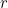,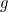, andvalues in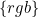to the 1/2.2 power. The more complicated, actual specifications for sRGB require this transformation: for each,, andcomponent of, let’s generically call it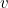, if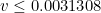, use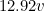, otherwise use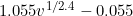. This can be done in Excel with the conditional =IF(v<0.0031308, 12.92*v, 1.055*v^(1/2.4)-0.055). The result of this will be true sRGB values in the range 0 to 1. Multiply them by 255 and round to the nearest integer to get the alternate range of 0 to 255, as used in Photoshop for example (in Excel: =ROUND(255 * IF(v<0.0031308, 12.92*v, 1.055*v^(1/2.4)-0.055), 0)). More information on this conversion process can be found at Bruce Lindbloom's highly informative website.

Thus, we now have a way to compute an sRGB triplet given a particular reflectance curve,. For the purposes of subtractive color mixture of two sRGB colors, if we know the reflectance curves corresponding to the two sRGB colors, we can perform the subtractive mix on the reflectance curves to arrive at another reflectance curve, and then compute the sRGB values from the mixture reflectance using the equation above. How is the subtractive mix of reflectances achieved? This is discussed next.